• +91 9971497814
• info@interviewmaterial.com

# Triangles EX-6.5 Interview Questions Answers

### Related Subjects

Question 1 :
Sides of triangles are given below. Determine which of them are right triangles. In case of a right triangle, write the length of its hypotenuse.

(i) 7 cm, 24 cm, 25 cm
(ii) 3 cm, 8 cm, 6 cm
(iii) 50 cm, 80 cm, 100 cm
(iv) 13 cm, 12 cm, 5 cm

Solution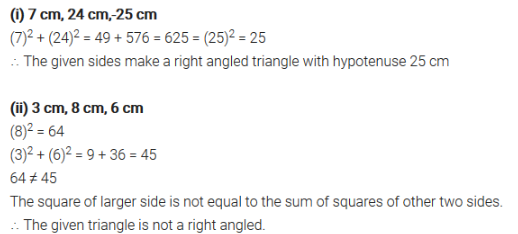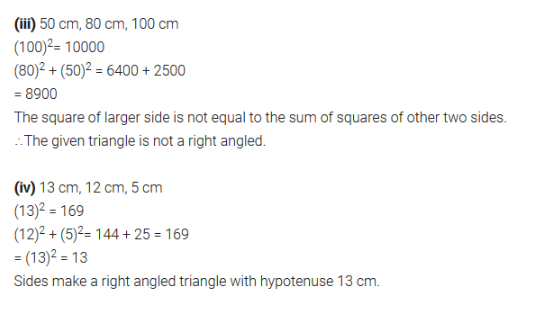Question 2 : PQR is a triangle right angled at P and M is apoint on QR such that PM QR. Show that PM2 = QM × MR.

Given, ΔPQR is right angled at P is a point onQR such that PM QR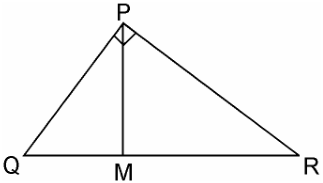We have to prove, PM2 =QM × MR

In ΔPQM, by Pythagoras theorem

PQ2 = PM2 + QM2

Or, PM2 = PQ2 –QM2 ……………………………..(i)

In ΔPMR, by Pythagoras theorem

PR2 = PM2 + MR2

Or, PM2 = PR2 –MR2 ………………………………………..(ii)

Adding equation, (i) and (ii), we get,

2PM2 = (PQ2 +PM2) – (QM2 + MR2)

= QR2 –QM2 – MR2        [ QR2 = PQ2 + PR2]

=(QM + MR)2 – QM2 – MR2

= 2QM × MR

PM2 = QM × MR

Question 3 :

In Figure, ABD is atriangle right angled at A and AC BD. Show that

Answer 3 : (i) AB_2 = BC × BD
(ii) AC_2 = BC × DC
(iii) AD_2 = BD × CD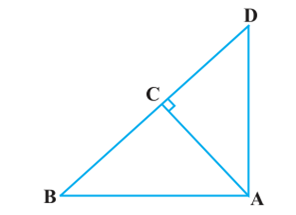Solution:

DAB = ACB (Each 90°)

ABD = CBA (Common angles)

ΔADB ~ ΔCAB [AA similarity criterion]

AB/CB = BD/AB

AB2 = CB × BD

Solution:

(ii) Let CAB = x

In ΔCBA,

CBA = 180° – 90° – x

CBA = 90° – x

= 90° – x

CDA = 180° – 90° – (90° – x)

CDA = x

In ΔCBA and ΔCAD, we have

CAB = CDA

ACB = DCA (Each 90°)

ΔCBA ~ ΔCAD [AAA similarity criterion]

AC/DC = BC/AC

AC2 =  DC × BC

Solution:

(iii) In ΔDCA and ΔDAB,

DCA = DAB (Each 90°)

ΔDCA ~ ΔDAB [AA similarity criterion]

DC/DA = DA/DA

Question 4 :

ABC is an isoscelestriangle right angled at C. Prove that AB2 = 2AC2 .

Given, ΔABC is an isosceles triangle rightangled at C.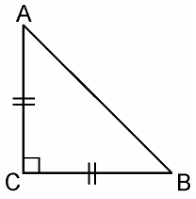In ΔACB, C = 90°

AC = BC (By isosceles triangle property)

AB2 = AC2 + BC2 [ByPythagoras theorem]

= AC2 + AC2 [Since,AC = BC]

AB2 = 2AC2

Question 5 :

ABC is an isoscelestriangle with AC = BC. If AB2 = 2AC2, provethat ABC is a right triangle.

Given, ΔABC is an isosceles triangle having AC= BC and AB2 = 2AC2In ΔACB,

AC = BC

AB2 = 2AC2

AB2 = AC+ AC2

= AC2 +BC[Since, AC = BC]

Hence, by Pythagoras theorem ΔABC isright angle triangle.

Question 6 :

ABC is anequilateral triangle of side 2a. Find each of its altitudes.

Given, ABC is an equilateral triangle of side2a.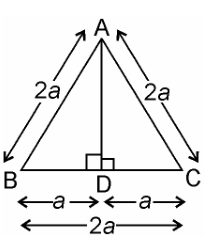AB = AC

Hence, BD = DC [by CPCT]

Question 7 :

Prove that the sumof the squares of the sides of rhombus is equal to the sum of the squares ofits diagonals.

Given, ABCDis a rhombus whose diagonals AC and BD intersect at O.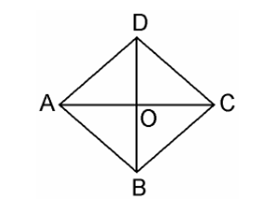We have to prove, as per the question,

AB+ BC+ CD2 +AD= AC+ BD2

Since, the diagonals of a rhombus bisect eachother at right angles.

Therefore, AO = CO and BO = DO

In ΔAOB,

AOB = 90°

AB2 = AO+ BO…………………….. (i) [By Pythagoras theorem]

Similarly,

DC2 = DO+ CO…………………….. (iii)

BC2 = CO+ BO…………………….. (iv)

Adding equations (i) + (ii) + (iii) + (iv), we get,

AB+ AD+ DC+ BC2 =2(AO+ BO+ DO+ CO2)

= 4AO+ 4BO[Since, AO =CO and BO =DO]

= (2AO)+(2BO)2 = AC+ BD2

AB+ AD+ DC+ BC2 =AC+ BD2

Hence, proved.

Question 8 : In the given figure, O is a point in theinterior of a triangle ABC, OD BC, OE AC and OF AB. Show that

Answer 8 : (i) OA_2 + OB_2 + OC_2 – OD_2 – OE_2 – OF_2 = AF_2 + BD_2 + CE_2
(ii) AF_2 + BD_2 + CE_2 = AE_2 + CD_2 + BF_2

_
_
_
_
_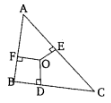_
_
_
_
_
_
_
_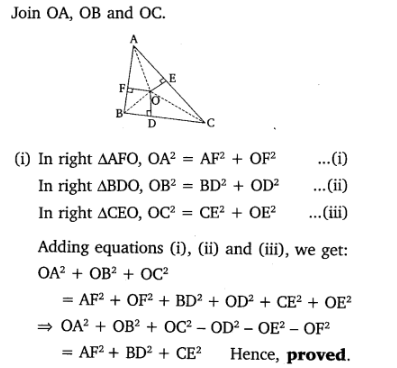_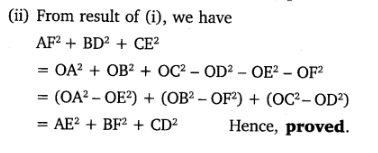_
_
_
_
_
_

Question 9 : A ladder 10 m long reaches a window 8 m abovethe ground. Find the distance of the foot of the ladder from base of the wall.

Given, a ladder 10 m long reaches a window 8 mabove the ground.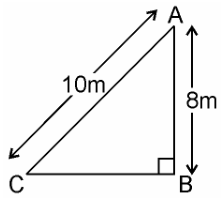Let BA be the wall and AC be the ladder,

Therefore, by Pythagoras theorem,

AC2 = AB2 +BC2

102 = 82 + BC2

BC= 100 – 64

BC= 36

BC = 6m

Therefore, the distance of the foot of theladder from the base of the wall is 6 m.

Question 10 :

A guy wire attachedto a vertical pole of height 18 m is 24 m long and has a stake attached to theother end. How far from the base of the pole should the stake be driven so thatthe wire will be taut?

Given, a guy wire attached to a vertical poleof height 18 m is 24 m long and has a stake attached to the other end.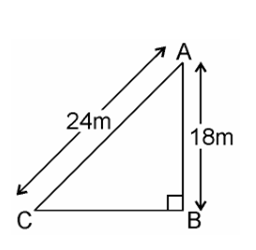Let AB be the pole and AC be the wire.

By Pythagoras theorem,

AC2 = AB2 +BC2

242 = 182 + BC2

BC= 576 – 324

BC= 252

BC = 6√7m

Therefore, the distance from the base is 6√7m.

Todays Deals### Triangles EX-6.5 Contributorskrishan

Name:
Email:

# Latest News# 9000 interview questions in different categories